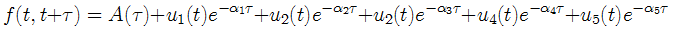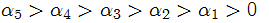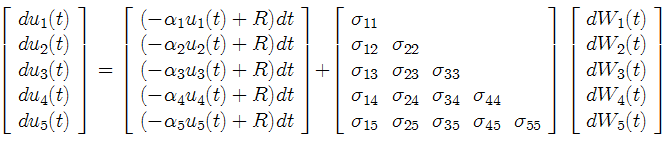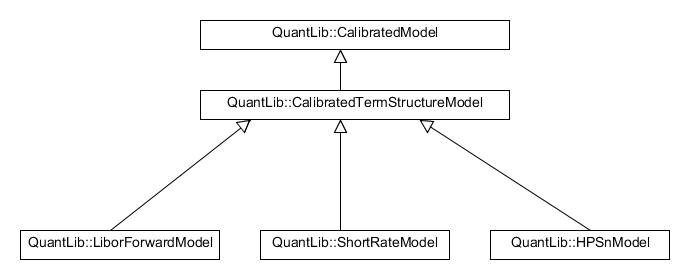﻿ Implementing the HPSn term-structure model in QuantLib: a working plan

# Implementing the HPSn term-structure model in QuantLib: a working plan

by Vasily Nekrasov. 20.04.2013

## Introduction

The HPSn model is a tractable multi-factor dynamic term-structure model developed by Michael Henseler, Christoph Peters and Roland Seydel, n stands for the number of factors.
It belongs to the class of Heath-Jarrow-Morton (HJM) Models.
In the original paper the authors particularly consider the case with five factors, so I will shortly explain the model for this case. This is just to make the explanations clear, as to implementation, the model should certainly be implemented in full generality.

In the HPS5 model the forward rates evolve (under the real-world measure) according to(1)
whereare fixed and the weights u(t) are stochastic and follow the Ornstein-Uhlenbeck process(2)
Note the separation of t and τ in (1)
Since the forward rates are observable, one can use (1) to fit a current yield curve. However, it is not a straightforward routine due to the no arbitrage conditions on term A(τ) (see the original paper).

Our goal is to implement the HPSn model in QuantLib conformably to the architecture of QuantLib. Obviosly we would like to (re)-use the building blocks already available in QuantLib.
We review the relevant parts of QuantLib and then suggest a plan for the implementation of the HPSn model.

## Term Structure Models and relevant classes in QuantLib

The HPSn belongs to the class of affine term structure models, which are probably the most popular term structure models. In QuantLib there is the basic (abstract) class QuantLib::AffineModel.
All three methods of this basis class, i.e. discount(), discountBond() and discountBondOption() must be implemented in inherited classes. Currently (v 1.21) the hierarchy of the affine models in QuantLib is as follows. According to the "status quo" it would be reasonable to derive the class QuantLib::HPSnModel directly from QuantLib::AffineModel (analogously to QuantLib::LiborForwardModel).

Another important class is the class of the term structure consistent models, i.e. the models that can perfectly reproduce a (current) yield curve. However, the HPSn model does not belong to this class. (At first glance it should since there is an affine term A(τ), which could have been chosen so that we perfectly fit f(t, t+τ) for any τ. But the term A(τ) must obey the no arbitrage conditions. Moreover, having the bond prices just for one trading day we cannot fit a yield curve for this day according to (1) because the term A(τ) depends on the covariance matrix of u1(t), ... , un(t) which can be determined only from time series of bond prices or forward rates. Finally the authors tend to work with A(τ) = const when it comes to model calibration.)
That's why we cannot integrate the HPSn in the realm of yield term structures. However, we do need to supply the pricing engines (see below) with a term structure. So we have to choose the "best" one among available. Nelson-Siegel-Svennson may be a good choice; though it does not perfectly match all bond prices but in terms of exponential basis functions it is similar to (1).
Note that if we could have dropped this "annoying" term A(τ) (we cannot due to the no arbitrage condition), we would just need to implement a new curve fitting method and then use it with QuantLib::FittedBondDiscountCurve and so stay "purely HPSn".

Last but not least we consider the class of calibrated models (I marked with red the term structure relevant parts). Again it seems to be reasonable to derive QuantLib::HPSnModel directly from QuantLib::CalibratedModel (again analogously to QuantLib::LiborForwardModel).

However, for a better manageability one probably should redesign the class hierarchy a little bit and introduce a class QuantLib::CalibratedTermStructureModel like thisOn the other hand it may be a multiplication of the entities without necessity since the business logic (i.e. calibrate() method) is implemented directly in the basis class QuantLib::CalibratedModel...
The calibration context is distinguished via Calibration Helpers. It might be surprising but we do not need to impelement any new calibration helpers. However, we do need to impelement the pricing engines. The hierarchy of the pricing engines got pretty unmanagable in QuantLib, even Doxygen failed to generate a pdf (yet after some tuning it did). Indeed, we need a separate pricing engine for (nearly) every model and every financial instrument. However, this approach is still probably the best because the implementations of the financial instruments and the models remain as independent as possible. It would be much worse to bloat the code of model classes with pricers for different instruments and even worser to implement the (model dependent) pricing directly in financial instruments. Finally, there are very many pricing engines but one can just purchase a larger monitor (or generate the graphs in a compacter way) :).

There is an analytic formula for the swaptions in the original paper. Thus we can (relatively quickly) calibrate the HPSn model to the available swaption prices and then use it to price the swaptions with other maturities. We need to implement additional pricing engines if we want to price other kind of derivatives.

## Sketch of classes and methods to implement

After a brief preliminary analysis we may figure out what we need to implement and we can already (re)use.
At minimum, we need the following (the new classes to implement are marked with dashed rectangles):The meaning of parameters volaMatrix_, alphas_, R_ and A_ should be clear from the equations (1) and (2). (According to the QuantLib coding style the names of the class member variables should end with "_"). As to the parameter b_, it is the market price of risk. The HPSn Model was developed to be suitable both for pricing (under the martingale measure) and risk management / portfolio optimisation (und the real-world measure). However, in QuantLib one distinctly tends to work under the martingale measures. Hence we should do so as well and switch to the real-world measure via the market price of risk. Such implementation is analogous to e.g. that of Vasicek model (in the implementation of the Vasicek model there is a parameter λ for the market price of risk).

The methods discount(), discountBond() and discountBondOption() are imposed by inheritance from QuantLib::AffineModel and must be implemented. Note that there are analytical formulae for the European call and put options on zerobonds (see original paper).
virtual void setParams(const Array& params) is implemented in QuantLib::CalibratedModel but we have complex parameters (volatility Matrix, vector of alphas), so we have to overwrite this method analogously to QuantLib::LiborForwardModel.

getSimulatedPath(unsigned long numberOfPathes, bool isUnderMartingaleMeasure) is an essential method. The model is multi-factor thus we generally should [be able to] price by means of the Monte Carlo simulation. (With trees and lattices that are so popular in QuantLib we would confront the curse of dimension). It is also essential for the risk management to be able to simulate the bond prices and/or forward rates. Monte-Carlo is still computationally intensive but we can gain from (currently ubiquitous) multi-core processors and simulate many paths at once. Moreover, we can even specify (e.g. by a C++ preprocessor flag) whether we should compile with GPU/CUDA in mind and if yes, simulate on a graphic card. It would be very nice feature: Luigi Ballabio himself admits that "the biggest single limitation right now is that we are moving towards a parallel processing, multi-core environment and Quantlib isn't really suited for that."
In favor of a multi-core simulation we also reject the Lazy Object Pattern (so beloved in QuantLib) and generate the new path(s) by every call. (Otherwise we would have to call calculate(unsigned long numberOfPathes) first then either getSimulatedPath(unsigned long numberOfPathes) carefully looking that numberOfPathes is the same in both calls or call getSimulatedPath() and check how many pathes there are...).

Last but not least, calibrateToHistoricalData() is necessary for the risk management applications and in particular for the determination of the market price of risk and calibration under the real-world measure. It is reasonable to implement this method directly within the model class. Introduction of auxiliary classes (something like QuantLib::CalibrationHelperForRealWorldMeasure analog to existing QuantLib::CalibrationHelper) would bring just mess, since the calibration to the historical data is very model-specific. Thus it should be tightly coupled with the model.
It is still an open question, which parameters calibrateToHistoricalData() should have. Most likely, we need several versions of this method: one accepting "raw" historical forward rates, other one accepting a vector of historical term structures, etc.

Obvously, we need an implementation for a multivariate Ornstein-Uhlenbeck process according to (2) but for this we already have all necessary "Lego bricks" in QuantLib. One way (probably not the optimal but working one) is to couple n univariate OU-Process with volatility matrix and the vector of alphas. Of course these parameters of a process should be tightly coupled with those of a model, what we can achieve by means of the Observer, Observable pattern.
Note that it probably might be easier to inherite not from QuantLib::JointStochasticProcess but directly from QuantLib::StochasticProcess. However, it would be a (slight) violation of the architecture.

Finally, the pricing engines are to implement analogously to those by other models. At minimum we need a pricing engine for swaptions, which are the most liquid derivatives. As soon as we have a swaption pricing engine, we can calibrate the model by means of QuantLib::SwaptionHelper.

Back to yetanotherquant.de